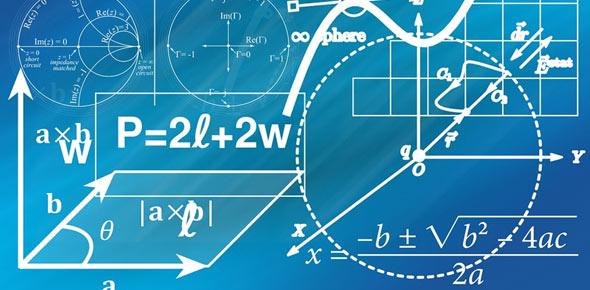# Distance Formula Quiz Question

10 Questions | Attempts: 297Settings• 1.
Find the distance between (2,2) and (6,2).
• 2.
Find the distance between (-1,3) and (2,-1).
• 3.
Find the distance between (-3,-2) and (5,4).
• 4.
Find the distance between (-6,-3) and (6,6).
• 5.
Find the distance between (-8,2) and (4,7).
• 6.
Find the distance between (-2,2) and (10,11).
• 7.
Find the distance between (-10,8) and (-2,-7).
• 8.
Find the distance between (-2,-10) and (-8,-2).
• 9.
Find the distance between (-5,-3) and (7,6).
• 10.
Find the distance between (-2,-10) and (10,6).Back to top